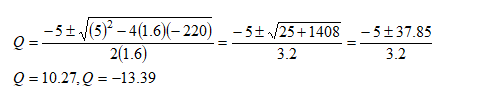In: Economics

# What is the profit maximizing level of output for a firm with the marginal cost function MC

What is the profit maximizing level of output for a firm with the marginal cost function MC = 1.6Q2-15Q+60 and a marginal revenue function MR = 280-20Q?

## Solutions

##### Expert Solution

Profit is maximized where;

Marginal revenue (MR) = Marginal cost (MC)

280-20Q = 1.6Q2-15Q+60

1.6Q2+5Q-220=0

Using quadratic formula to solve for Q

a=1.6, b=5, c=-220There cannot be can’t have negative output.

Therefore, Profit maximising level of output is Q = 10.27.

There cannot be can’t have negative output. Therefore, Profit maximising level of output is Q = 10.27.